## Example Questions

### Example Question #135 : Integers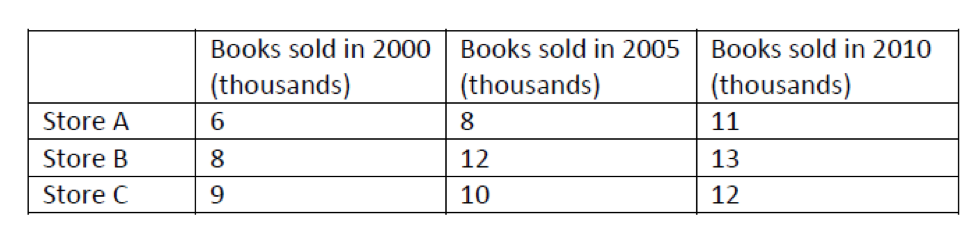Which store had the greatest increase in thousands of book sales between 2000 and 2010?

Store A

Stores A and B tie

Store C

Stores B and C tie

Store B

Stores A and B tie

Explanation:

Calculate the difference in thousands of sales for each store individually:

Store A: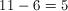thousand

Store B: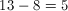thousand

Store C: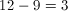thousand

Stores A and B both had a 5000-book increase in sales, so A and B tie.

### Example Question #11 : Operations

Choose the answer below which best solves the following equation: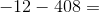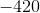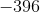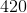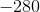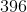Explanation: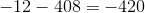If it helps, for this problem, think about starting at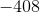and moving twelve places in a negative direction away from zero.

Also you can think to add 408 with 12 and then multiply that number by a negative one. Starting with the ones place eight plus two gives ten so we keep the zero in the ones place and carry the one to the tens place. Then we add one plus one to give us a two in the tens column. Next we have a four in the hundreds place. This gives us 420. Now we multiply this by negtive one to get our final answer of -420.

### Example Question #137 : Integers

Choose the answer which best solves the equation below: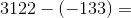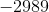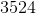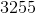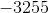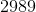Explanation: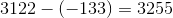Whenever you subtract a negative number, you flip the sign and add: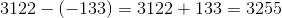Tired of practice problems?

Try live online GRE prep today.